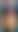# Join us to combat climate change & rewild the worldself.__wrap_n=self.__wrap_n||(self.CSS&&CSS.supports("text-wrap","balance")?1:2);self.__wrap_b=(e,t,r)=>{let a=(r=r||document.querySelector(`[data-br="\${e}"]`)).parentElement,l=e=>r.style.maxWidth=e+"px";r.style.maxWidth="";let n=a.clientWidth,o=a.clientHeight,u=n/2-.25,i=n+.5,s;if(n){for(l(u),u=Math.max(r.scrollWidth,u);u+1<i;)l(s=Math.round((u+i)/2)),a.clientHeight===o?i=s:u=s;l(i*t+n*(1-t))}r.__wrap_o||"undefined"!=typeof ResizeObserver&&(r.__wrap_o=new ResizeObserver(()=>{self.__wrap_b(0,+r.dataset.brr,r)})).observe(a)};self.__wrap_n!=1&&self.__wrap_b(":Rcddclda:",1)

## What is a Carbon Jacked membership?self.__wrap_n=self.__wrap_n||(self.CSS&&CSS.supports("text-wrap","balance")?1:2);self.__wrap_b=(e,t,r)=>{let a=(r=r||document.querySelector(`[data-br="\${e}"]`)).parentElement,l=e=>r.style.maxWidth=e+"px";r.style.maxWidth="";let n=a.clientWidth,o=a.clientHeight,u=n/2-.25,i=n+.5,s;if(n){for(l(u),u=Math.max(r.scrollWidth,u);u+1<i;)l(s=Math.round((u+i)/2)),a.clientHeight===o?i=s:u=s;l(i*t+n*(1-t))}r.__wrap_o||"undefined"!=typeof ResizeObserver&&(r.__wrap_o=new ResizeObserver(()=>{self.__wrap_b(0,+r.dataset.brr,r)})).observe(a)};self.__wrap_n!=1&&self.__wrap_b(":R1cldclda:",1)

The world's coolest sustainable membership - that plants trees, funds projects that restore nature and reduces your carbon footprint.## Reasons to joinself.__wrap_n=self.__wrap_n||(self.CSS&&CSS.supports("text-wrap","balance")?1:2);self.__wrap_b=(e,t,r)=>{let a=(r=r||document.querySelector(`[data-br="\${e}"]`)).parentElement,l=e=>r.style.maxWidth=e+"px";r.style.maxWidth="";let n=a.clientWidth,o=a.clientHeight,u=n/2-.25,i=n+.5,s;if(n){for(l(u),u=Math.max(r.scrollWidth,u);u+1<i;)l(s=Math.round((u+i)/2)),a.clientHeight===o?i=s:u=s;l(i*t+n*(1-t))}r.__wrap_o||"undefined"!=typeof ResizeObserver&&(r.__wrap_o=new ResizeObserver(()=>{self.__wrap_b(0,+r.dataset.brr,r)})).observe(a)};self.__wrap_n!=1&&self.__wrap_b(":Rctdclda:",1)### 1. Plant trees

& Rewild the world### 2. Improve lives

Through epic projects that combat climate change### 3. Connect with nature

Be inspired to get into nature & adventure

### P.S the planet is burning

What are you waiting for?## Choose your membershipself.__wrap_n=self.__wrap_n||(self.CSS&&CSS.supports("text-wrap","balance")?1:2);self.__wrap_b=(e,t,r)=>{let a=(r=r||document.querySelector(`[data-br="\${e}"]`)).parentElement,l=e=>r.style.maxWidth=e+"px";r.style.maxWidth="";let n=a.clientWidth,o=a.clientHeight,u=n/2-.25,i=n+.5,s;if(n){for(l(u),u=Math.max(r.scrollWidth,u);u+1<i;)l(s=Math.round((u+i)/2)),a.clientHeight===o?i=s:u=s;l(i*t+n*(1-t))}r.__wrap_o||"undefined"!=typeof ResizeObserver&&(r.__wrap_o=new ResizeObserver(()=>{self.__wrap_b(0,+r.dataset.brr,r)})).observe(a)};self.__wrap_n!=1&&self.__wrap_b(":Rl5dclda:",1)### Carbon Cub

1. Plant 1 tree a month 🌲
2. Fund amazing projects that restore nature & improve lives 🌍
3. Reduce your carbon footprint with our calculator & advice 👣 📉

And much more

Become a cub### Carbon Jacked

1. Plant 10 trees a month 🌲
2. Majorly fund amazing projects that restore nature & improve lives 🌍
3. Reduce your carbon footprint with our calculator & advice 👣 📉

And much more

Get jacked
Can’t afford a membership?

## Projects your membership supportsself.__wrap_n=self.__wrap_n||(self.CSS&&CSS.supports("text-wrap","balance")?1:2);self.__wrap_b=(e,t,r)=>{let a=(r=r||document.querySelector(`[data-br="\${e}"]`)).parentElement,l=e=>r.style.maxWidth=e+"px";r.style.maxWidth="";let n=a.clientWidth,o=a.clientHeight,u=n/2-.25,i=n+.5,s;if(n){for(l(u),u=Math.max(r.scrollWidth,u);u+1<i;)l(s=Math.round((u+i)/2)),a.clientHeight===o?i=s:u=s;l(i*t+n*(1-t))}r.__wrap_o||"undefined"!=typeof ResizeObserver&&(r.__wrap_o=new ResizeObserver(()=>{self.__wrap_b(0,+r.dataset.brr,r)})).observe(a)};self.__wrap_n!=1&&self.__wrap_b(":Rlddclda:",1)### Tree planting in the peak district### Preventing deforestation & empowering local communities in tanzania### Restoring peatland & capturing carbon in the Peak district### Planting mangroves, creating jobs & saving lives in Madagascar### Helping refugees & lowering emissions in chad with solar cookers

See full deets on our projects

## Sustainable clothingself.__wrap_n=self.__wrap_n||(self.CSS&&CSS.supports("text-wrap","balance")?1:2);self.__wrap_b=(e,t,r)=>{let a=(r=r||document.querySelector(`[data-br="\${e}"]`)).parentElement,l=e=>r.style.maxWidth=e+"px";r.style.maxWidth="";let n=a.clientWidth,o=a.clientHeight,u=n/2-.25,i=n+.5,s;if(n){for(l(u),u=Math.max(r.scrollWidth,u);u+1<i;)l(s=Math.round((u+i)/2)),a.clientHeight===o?i=s:u=s;l(i*t+n*(1-t))}r.__wrap_o||"undefined"!=typeof ResizeObserver&&(r.__wrap_o=new ResizeObserver(()=>{self.__wrap_b(0,+r.dataset.brr,r)})).observe(a)};self.__wrap_n!=1&&self.__wrap_b(":R2dldclda:",1)Kit yourself out in Carbon Jacked clothing

Shop

## Carbon Jacked projectsself.__wrap_n=self.__wrap_n||(self.CSS&&CSS.supports("text-wrap","balance")?1:2);self.__wrap_b=(e,t,r)=>{let a=(r=r||document.querySelector(`[data-br="\${e}"]`)).parentElement,l=e=>r.style.maxWidth=e+"px";r.style.maxWidth="";let n=a.clientWidth,o=a.clientHeight,u=n/2-.25,i=n+.5,s;if(n){for(l(u),u=Math.max(r.scrollWidth,u);u+1<i;)l(s=Math.round((u+i)/2)),a.clientHeight===o?i=s:u=s;l(i*t+n*(1-t))}r.__wrap_o||"undefined"!=typeof ResizeObserver&&(r.__wrap_o=new ResizeObserver(()=>{self.__wrap_b(0,+r.dataset.brr,r)})).observe(a)};self.__wrap_n!=1&&self.__wrap_b(":R2lldclda:",1)Check out our amazing climate projects

Projects

## Carbon Jacked for businessself.__wrap_n=self.__wrap_n||(self.CSS&&CSS.supports("text-wrap","balance")?1:2);self.__wrap_b=(e,t,r)=>{let a=(r=r||document.querySelector(`[data-br="\${e}"]`)).parentElement,l=e=>r.style.maxWidth=e+"px";r.style.maxWidth="";let n=a.clientWidth,o=a.clientHeight,u=n/2-.25,i=n+.5,s;if(n){for(l(u),u=Math.max(r.scrollWidth,u);u+1<i;)l(s=Math.round((u+i)/2)),a.clientHeight===o?i=s:u=s;l(i*t+n*(1-t))}r.__wrap_o||"undefined"!=typeof ResizeObserver&&(r.__wrap_o=new ResizeObserver(()=>{self.__wrap_b(0,+r.dataset.brr,r)})).observe(a)};self.__wrap_n!=1&&self.__wrap_b(":R2tldclda:",1)